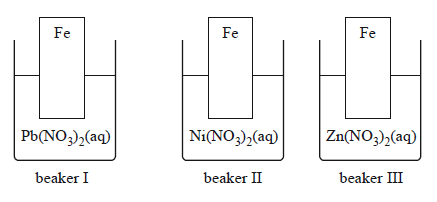General (2013 VCE) 1)Consider the following. Calculate the pressure exerted by 6.9 g of argon in a 0.07500 L container at 11.5 °C. The number of significant figures that should be expressed in the answer is A. 2 B. 3 C. 4 D. 5 Solution2) In an experiment, 5.85 g of ethanol was ignited with 14.2 g of oxygen. i. Write an equation for the complete combustion of ethanol. Solutionii) Which reagent is in excess? Calculate the amount, in moles, of the reagent identified as being in excess. SolutionExaminer's comment iii) Genetically modified yeast is used to convert xylose, C5H10O5, another monosaccharide found in plant fibres, to ethanol. 3C5H10O5(aq) => 5C2H5OH(l) + 5CO2(g) In a trial, 1.00 kg of pure xylose is completely converted to ethanol and carbon dioxide. Calculate the volume, in mL, of ethanol that is produced. Note: The density of ethanol is 0.785 g mL–1. Examiner's comment Solutioniv) Determine the volume of carbon dioxide gas at 20.0 °C and 750.0 mm pressure produced by the xylose. Solution3) Two identical flasks, A and B, contain, respectively, 5.0 g of N2 gas and 14.4 g of an unknown gas. The gases in both flasks are at standard laboratory conditions (SLC). The gas in flask B is A. H2 B. SO2 C. HBr D. C4H10 Solution4) Which one of the following reactions is a redox reaction? A. 2Al(s) + 3Cl2(g) => 2AlCl3(s) B. Pb2+(aq) + 2Cl–(aq) => PbCl2(s) C. NaOH(aq) + HCl(aq) => NaCl(aq) + H2O(l) D. CH3OH(l) + HCOOH(l) => COOCH3(l) + H2O(l) Solution5) The following is a student's summary of catalysts. It contains some correct and incorrect statements. a. A catalyst increases the rate of a reaction. b. All catalysts are solids. c. The mass of a catalyst is the same before and after the reaction. d. A catalyst lowers the enthalpy change of a reaction, enabling more particles to have sufficient energy to successfully react. e. A catalyst increases the value of the equilibrium constant, thus favouring the extent of the forward reaction, resulting in a greater yield of product. f. All catalysts align the reactant particles in an orientation that is favourable for a reaction to occur. g. The effectiveness of a metal catalyst is not dependent upon its surface area. h. Enzymes are biological catalysts that catalyse a specific biochemical reaction once only. i. The effectiveness of an enzyme is independent of temperature. a) Identify two correct statements. Solution b) Evaluate the student's summary by identifying three incorrect statements. In each case, explain why it is incorrect. Solution6) Three beakers, each containing an iron strip and a 1.0 M solution of a metal salt, were set up as follows.A reaction will occur in beaker(s) A. I and II only. B. I and III only. C. II and III only. D. III only. Solution